# Markov chain

2010 Mathematics Subject Classification: Primary: 60J10 Secondary: 60J27 [MSN][ZBL]

A Markov process with finite or countable state space. The theory of Markov chains was created by A.A. Markov who, in 1907, initiated the study of sequences of dependent trials and related sums of random variables [M].

Let the state space be the set of natural numbers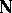or a finite subset thereof. Letbe the state of a Markov chain at time. The fundamental property of a Markov chain is the Markov property, which for a discrete-time Markov chain (that is, whentakes only non-negative integer values) is defined as follows: For any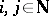, any non-negative integers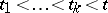and any natural numbers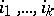, the equality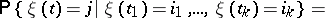(1)holds.

The Markov property (1) can be formulated as follows. The timeand the related events of the form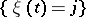are called the "present" of the process; events determined by the values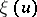,, are called the "past" of the process; events determined by,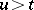, are called the "future" of the process. Then (1) is equivalent to the following: For anyand fixed "present"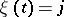, any "past" eventand "future" eventare conditionally independent, given the present. That is,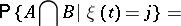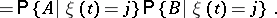In the probabilistic description of a Markov chain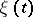the transition probabilities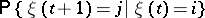(2)

play a major role. When (2) does not depend on, the Markov chain is called homogeneous (in time); otherwise it is called non-homogeneous. Only homogeneous Markov chains are considered below. Let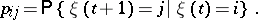The matrix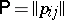with entries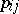is called the matrix of transition probabilities. The probability of an arbitrary trajectory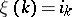,, is given by the transition probabilitiesand the initial distributionas follows: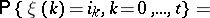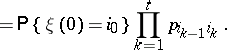Along with the transition probabilities, in a Markov chain the-step transition probabilities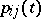are also discussed:These transition probabilities satisfy the Kolmogorov–Chapman equationUsing transition probabilities it is possible to give the following classification of states. Two statesandare called communicating if there are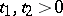such that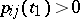and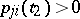. A stateis called inessential if there is a statesuch that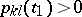for some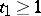and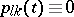for all. All remaining states are called essential. Thus, the complete state space of a Markov chain decomposes into inessential and essential states. The set of all essential states decomposes into disjoint classes of communicating states, such that any two states from one class communicate with each other, and for any two states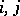from distinct classes,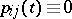,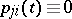. A Markov chain for which all states belong to one class of communicating states is called non-decomposable (cf. Markov chain, non-decomposable); otherwise the Markov chain is called decomposable (see Markov chain, decomposable). If the state space is finite, then its decomposition into these classes determines, to a great extent, the asymptotic properties of the Markov chain. For example, for a finite non-decomposable Markov chain the limit(3)

where, always exists. If, in addition, the Markov chain is aperiodic, that is, if for some, for alland all statesand,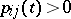(see also Markov chain, periodic), then there is the stronger result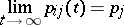(4)

If the state space of a Markov chain is countable, then its asymptotic properties depend on more subtle properties of the classes of communicating states. The series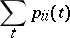(5)

simultaneously diverge or converge for all states of a given class. A class of states is called recurrent if for any stateof the class the series (5) diverges and non-recurrent if (5) converges. In a recurrent class, with probability 1 a Markov chain returns to any of its states, in a non-recurrent class the probability of recurrence is less than 1. If the mean return time in a recurrent class is finite, then the class is called positive; otherwise it is called zero (see Markov chain, class of positive states of a; Markov chain, class of zero states of a). Ifandbelong to the same class of positive states, then the limit (3) exists, and in the aperiodic case the limit (4) exists. Ifbelongs to a class of zero states or is inessential, then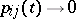as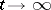.

Let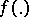be a real-valued function defined on the states of a Markov chain. If the Markov chain is non-decomposable and its states form a positive class, then for the sum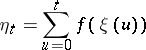the central limit theorem holds: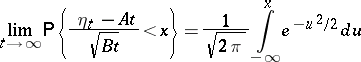(6)

for someand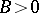. For (6) to hold it is sufficient to require in addition that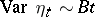,, and.

Iftakes any value in, then the chain is called a continuous-time Markov chain, defined in a similar way using the Markov property (1). Usually, for a continuous-time Markov chain one additionally requires the existence of finite right derivatives, called the transition probability densities. For a finite continuous-time Markov chain, from the Kolmogorov–Chapman equation one obtains the Kolmogorov differential equations(7)

and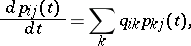(8)

with the initial conditions, where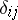is the Kronecker symbol. Under additional assumptions (7) and (8) also hold for countable Markov chains.

If a continuous-time Markov chain has a stationary distribution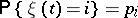(that is, the distribution ofdoes not depend on the time), then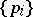satisfies the system of linear equations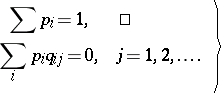(9)

Markov chains have been widely used in the solution of various applied problems. For example, in queueing theory, for the calculation of the distribution of the number of busy lines in an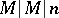system with refusals (that is, in a system comprisinglines with Poisson flow of requests and exponential law of service time) a finite continuous-time Markov chain with states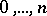and the following transition probability densities is used: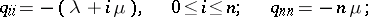(here,is the intensity of the Poisson flow of requests and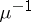is the mean service time). Using (9) the stationary distribution of the number of busy lines in this case can be determined as: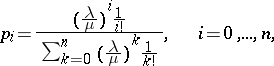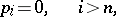which is called the Erlang distribution.